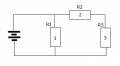# Where did I go wrong?

#### Loki4000

Joined Sep 29, 2011
22
Greetings,

Recently I try to calculate simple circuit:First I calculated resistances on the right corner

1/((1/1)+(1/3)) = rx1

then

1/((1/rx1)+(1/2)) = rx2

then

1/((1/rx2)+(1/1.5)) = rx3

then

1+rx3+1 = rxfinal

I enden up with 2.4

but according to this programm total resistance must be: 2970,29703ohm
(V/I)I want to know where did I go wrong in my calculations?

#### DerStrom8

Joined Feb 20, 2011
2,390
Instead of using the 1/((1/R1)+(1/R2)....), try starting at the right hand side (right-most resistors are in parallel, see?) and try (R1*R2)/(R1+R2).

The first formula is only used to find the total resistance of a bunch of resistors only in parallel.

Der Strom

#### RiJoRI

Joined Aug 15, 2007
536
I'm lazy. Why memorize two formulae when 1 will do?

While in tech school I was told that the safest way of doing this is to redraw the circuit every time you collapse a branch into its equivalent. When you collapse R4&R5, and redraw the circuit, you will see where your problem lies. Remember, do the serial calculations before the parallel.

--Rich

#### DerStrom8

Joined Feb 20, 2011
2,390
I'm lazy. Why memorize two formulae when 1 will do?
Unfortunately, the one formula you memorized wouldn't work. You'll need the other one for this problem. As you said, you need to collapse the resistors down into their equivalent, and for that you will need (RxRy)/(Rx+Ry).

#### Loki4000

Joined Sep 29, 2011
22
Instead of using the 1/((1/R1)+(1/R2)....), try starting at the right hand side (right-most resistors are in parallel, see?) and try (R1*R2)/(R1+R2).

The first formula is only used to find the total resistance of a bunch of resistors only in parallel.

Der Strom
Thanx for the reply, but now I am even more confused.

Can you show me what you mean using real numbers?

P.S. I already try starting at the right hand side. First I calculate total resistance of 1 and 3 kOHM resistor using 1/((1/1)+(1/3)) = rx1 , then treating answer as one new resistor plug it back and calculate this new resistor with 2kOHM resistor... and so on untill you move from right back to left, to voltage source. At least that's how I was told its supposed to be done. But double check on circuit calculating problem show that my calculation was wrong...

#### DerStrom8

Joined Feb 20, 2011
2,390
Thanx for the reply, but now I am even more confused.

Can you show me what you mean using real numbers?

P.S. I already try starting at the right hand side. First I calculate total resistance of 1 and 3 kOHM resistor using 1/((1/1)+(1/3)) = rx1 , then treating answer as one new resistor plug it back and calculate this new resistor with 2kOHM resistor... and so on untill you move from right back to left, to voltage source. At least that's how I was told its supposed to be done. But double check on circuit calculating problem show that my calculation was wrong...
Ok, let's look at the following circuit for this example. It is a simplified version of yours, but you solve it the same way:What is the first thing you notice about R2 and R3?

If you said that they're in series, you're right. So just add them up to get their equivalent resistance:

R2+R3 = 2+3 = 5 ohms. Let's call this value R' (R prime).

Now that you have the equivalent resistance of R2 and R3, you substitute in a 5 ohm resistor for them.

Now what do you notice about R1 and R'?

If you said that they're in parallel, then you're right again. So use the formula (RxRy)/(Rx+Ry) to find the equivalent resistance of both of them together.

(RxRy)/(Rx+Ry) = (R1*R')/(Rx+R') = (1*5)/(1+5) = 5/6 ohms. So that would be your Rt for this circuit. Now, if you simply put on more "stages", then after doing this, you would end up with a circuit looking very much like the one you started with, only there would be one less "stage". You would simply repeat the process over again.

Does this help?

Der Strom

#### RiJoRI

Joined Aug 15, 2007
536
unfortunately, the one formula you memorized wouldn't work. You'll need the other one for this problem. As you said, you need to collapse the resistors down into their equivalent, and for that you will need (rxry)/(rx+ry).
r1 = 1k
r2 = 2k

r1 || r2

1/((1/1000)+(1/2000))= 666.6666666667

(1000*2000)/(1000+2000)= 666.6666666667

#### DerStrom8

Joined Feb 20, 2011
2,390
r1 = 1k
r2 = 2k

r1 || r2

1/((1/1000)+(1/2000))= 666.6666666667

(1000*2000)/(1000+2000)= 666.6666666667
Whoops, sorry. Thanks Rich, you're right. That formula will work, but you just need to be careful of the values. If it is in kilohms, you have to scale the result, or convert to ohms. I have a feeling that may have been your mistake--you didn't scale it properly.

#### Loki4000

Joined Sep 29, 2011
22
Thanx for replies, but I figured the problem, turn out that after calculating 1 and 3 kOHM resistors and treating this sum as separate resistors, I add this separate resistor to 2 kOHM resistor in paralel rather than in series.

#### t06afre

Joined May 11, 2009
5,934
If it is in kilohms, you have to scale the result, or convert to ohms. I have a feeling that may have been your mistake--you didn't scale it properly.
This was somewhat cryptic to me. Can you elaborate?